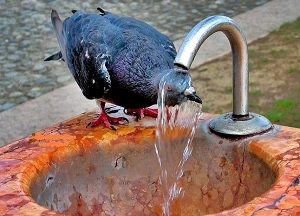Random Number, string, password In C#

Brian
Participant
18 Points
8 Posts

Introduction

The Random class of .NET class library provides functionality to generate random numbers in C#.
The Random class constructors have two overloaded forms. It takes either no value or it takes a seed value.

Details

The Random class has three public methods - Next, NextBytes, and NextDouble. The Next method returns a random number, NextBytes returns an array of bytes filled with random numbers, and NextDouble returns a random number between 0.0 and 1.0.

The following code returns a random number.

``int randomNum = random.Next();``

The following code returns a random number less than 1000.

``int randomNum = random.Next(1000);``

Example

The following code returns a random number between the min and the max range.

``// Generate a random number between two numbers public int RandomNumber(int min, int max) {   Random random = new Random();   return random.Next(min, max); }``

You can even combine the two methods - RandomNumber and RandomString to generate a combination of random string and numbers.

``// Generate a random string with a given size public string RandomString(int size, bool lowerCase) {   StringBuilder builder = new StringBuilder();   Random random = new Random();   char ch;   for (int i = 0; i < size; i++)   {     ch = Convert.ToChar(Convert.ToInt32(Math.Floor(26 * random.NextDouble() + 65)));     builder.Append(ch);   }   if (lowerCase)     return builder.ToString().ToLower();   return builder.ToString(); }``

The following code generates a password of length 10 with first 4 letters lowercase, next 4 letters numbers, and last 2 letters as uppercase.

``// Generate a random password public string RandomPassword() {   StringBuilder builder = new StringBuilder();   builder.Append(RandomString(4, true));   builder.Append(RandomNumber(1000, 9999));   builder.Append(RandomString(2, false));   return builder.ToString(); } ``